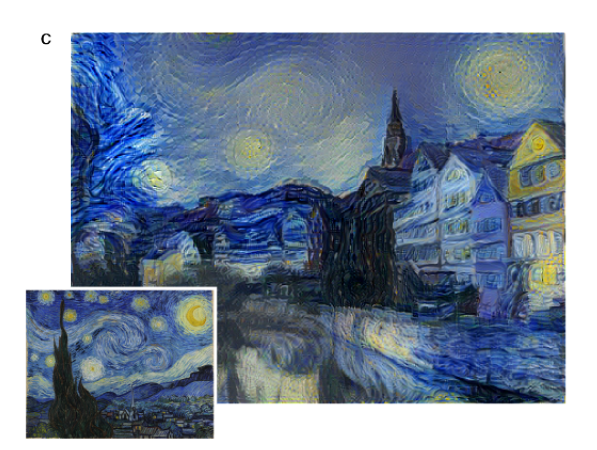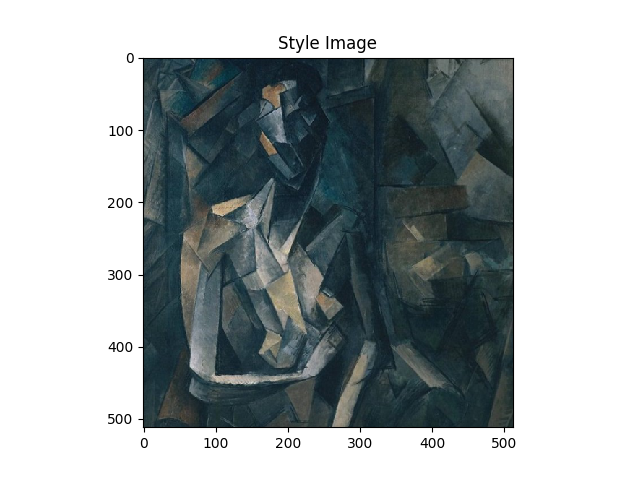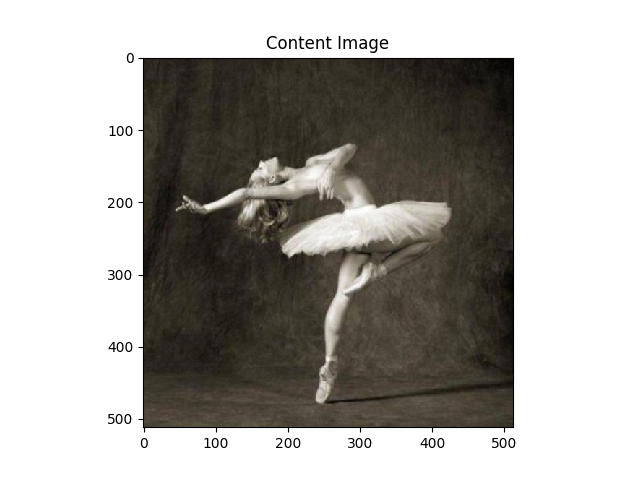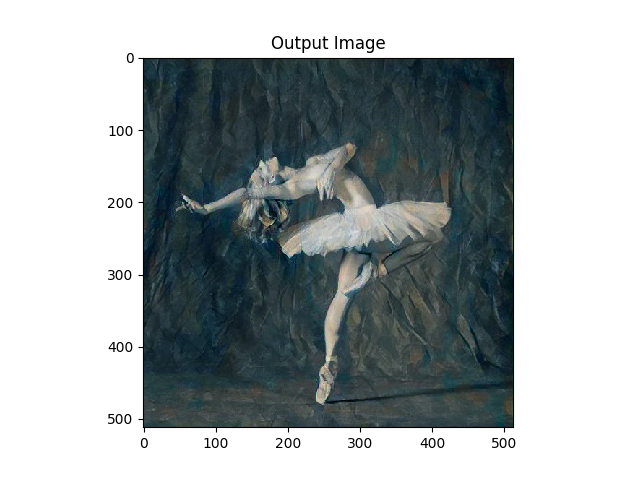# Neural Style Transfer

Posted by lili on

## 原理

Neural Style Transfer认为画家的作品包含两部分内容——内容(Content)和风格(Style)，而拍摄的照片只有内容。我们人类是很容易可以识别一幅画的内容同时也能识别其风格，也就是说我们可以把一幅画的内容和风格分割开来。$\mathcal{L}_{content}(\vec{p}, \vec{x}, l)=\frac{1}{2}\sum_{i,j} (F^l_{ij}-P^l_{ij})^2 \\ \mathcal{L}_{content}(\vec{p}, \vec{x}) =\sum_l \mathcal{L}_{content}(\vec{p}, \vec{x}, l)$

$G^l_{ij}=\sum_k F^l_{ik}F^l_{jk}$

Gram矩阵$G^l$的大小$N_l \times N_l$，计算相关(Correlation)是统计和信号处理中的常见技巧。有了$G^l$之后，我们也可以定义两个照片风格的Loss：

$E_l=\frac{1}{4N_l^2M_l^2} \sum_{i,j}(G^l_{ij}-A^l_{ij})^2 \\ \mathcal{L}_{style}=\sum_l E_l$

$\mathcal{L}_{total}=\alpha \mathcal{L}_{content} + \beta \mathcal{L}_{style}$

## 代码

Neural Style Transfer有很多开源实现，比较流行的包括jcjohnson的Torch/lua实现Anishathalye的Tensorflow实现。为了简单，我们使用PyTorch Tutorial的例子，Tutorial的作者是Alexis Jacq和Winston Herring，感谢他们授权本书使用。

### 导入package

from __future__ import print_function

import torch
import torch.nn as nn
import torch.nn.functional as F
import torch.optim as optim

from PIL import Image
import matplotlib.pyplot as plt

import torchvision.transforms as transforms
import torchvision.models as models

import copy


device = torch.device("cuda" if torch.cuda.is_available() else "cpu")


### 加载图片

# 如果没有GPU，选择较小的尺寸。
imsize = 512 if torch.cuda.is_available() else 128  # use small size if no gpu

transforms.Resize(imsize),  # 缩放
transforms.ToTensor()])  # 变成torch tensor

image = Image.open(image_name)
# 必须有个batch维度，因此用unsqueeze(0)增加一个batch维度。
# 如果是CPU，那么loader得到的是(3, 128, 128)，unsqueeze之后变成(1, 3, 128, 123)
return image.to(device, torch.float)



unloader = transforms.ToPILImage()  # 为了显示转换成PIL image

plt.ion()

def imshow(tensor, title=None):
image = tensor.cpu().clone()  # 因为显示是需要把batch去掉，为了避免修改，我们clone一份。
image = image.squeeze(0)  # 去掉batch维度
plt.imshow(image)
if title is not None:
plt.title(title)
plt.pause(0.001)  # 等一下以便plot更新

plt.figure()
imshow(style_img, title='Style Image')

plt.figure()
imshow(content_img, title='Content Image')### 损失函数

class ContentLoss(nn.Module):
def __init__(self, target):
super(ContentLoss, self).__init__()
self.target = target.detach()

def forward(self, input):
self.loss = F.mse_loss(input, self.target)
return input


StyleLoss也是类似的，只不过计算更加复杂一点，需要计算Gram矩阵。我们首先来看它的代码：

def gram_matrix(input):
a, b, c, d = input.size()  # a=batch size(=1)
# b=feature map的数量
# (c,d)=dimensions of a f. map (N=c*d)

features = input.view(a * b, c * d)  # resise F_XL into \hat F_XL

G = torch.mm(features, features.t())  # compute the gram product

# we 'normalize' the values of the gram matrix
# by dividing by the number of element in each feature maps.
return G.div(a * b * c * d)


Gram矩阵其实就是矩阵乘以它的转置，输入input可能是(1, 64, 512, 512)，分布表示batch，number_featuremaps, width, height。我们首先要把它reshape成(1x64, 512x512)，然后用features乘以它自己的转置，最后需要除以元素的个数，注意，这里和论文有点不同，论文是除以$4N_l^2M_l^2=4 \times 64^2 \times 512^4$。不过这并不重要，这只是一个常量，因为后面我们还会乘以一个StyleLoss的权重。

class StyleLoss(nn.Module):

def __init__(self, target_feature):
super(StyleLoss, self).__init__()
self.target = gram_matrix(target_feature).detach()

def forward(self, input):
G = gram_matrix(input)
self.loss = F.mse_loss(G, self.target)
return input


### 导入模型

cnn = models.vgg19(pretrained=True).features.to(device).eval()


cnn_normalization_mean = torch.tensor([0.485, 0.456, 0.406]).to(device)
cnn_normalization_std = torch.tensor([0.229, 0.224, 0.225]).to(device)

class Normalization(nn.Module):
def __init__(self, mean, std):
super(Normalization, self).__init__()
# 把mean和std reshape成 [C x 1 x 1]
# 输入图片是 [B x C x H x W].
self.mean = torch.tensor(mean).view(-1, 1, 1)
self.std = torch.tensor(std).view(-1, 1, 1)

def forward(self, img):
return (img - self.mean) / self.std


Sequential(
(0): Conv2d(3, 64, kernel_size=(3, 3), stride=(1, 1), padding=(1, 1))
(1): ReLU(inplace)
(2): Conv2d(64, 64, kernel_size=(3, 3), stride=(1, 1), padding=(1, 1))
(3): ReLU(inplace)
(4): MaxPool2d(kernel_size=2, stride=2, padding=0, dilation=1, ceil_mode=False)
(5): Conv2d(64, 128, kernel_size=(3, 3), stride=(1, 1), padding=(1, 1))
(6): ReLU(inplace)
(7): Conv2d(128, 128, kernel_size=(3, 3), stride=(1, 1), padding=(1, 1))
(8): ReLU(inplace)
(9): MaxPool2d(kernel_size=2, stride=2, padding=0, dilation=1, ceil_mode=False)
....
(33): ReLU(inplace)
(34): Conv2d(512, 512, kernel_size=(3, 3), stride=(1, 1), padding=(1, 1))
(35): ReLU(inplace)
(36): MaxPool2d(kernel_size=2, stride=2, padding=0, dilation=1, ceil_mode=False)
)


content_layers_default = ['conv_4']
style_layers_default = ['conv_1', 'conv_2', 'conv_3', 'conv_4', 'conv_5']

def get_style_model_and_losses(cnn, normalization_mean, normalization_std,
style_img, content_img,
content_layers=content_layers_default,
style_layers=style_layers_default):
cnn = copy.deepcopy(cnn)

normalization = Normalization(normalization_mean, normalization_std).to(device)

content_losses = []
style_losses = []

model = nn.Sequential(normalization)

i = 0  # increment every time we see a conv
for layer in cnn.children():
if isinstance(layer, nn.Conv2d):
i += 1
name = 'conv_{}'.format(i)
elif isinstance(layer, nn.ReLU):
name = 'relu_{}'.format(i)
layer = nn.ReLU(inplace=False)
elif isinstance(layer, nn.MaxPool2d):
name = 'pool_{}'.format(i)
elif isinstance(layer, nn.BatchNorm2d):
name = 'bn_{}'.format(i)
else:
raise RuntimeError('Unrecognized layer: {}'
.format(layer.__class__.__name__))

if name in content_layers:
target = model(content_img).detach()
content_loss = ContentLoss(target)
content_losses.append(content_loss)

if name in style_layers:
target_feature = model(style_img).detach()
style_loss = StyleLoss(target_feature)
style_losses.append(style_loss)

for i in range(len(model) - 1, -1, -1):
if isinstance(model[i], ContentLoss) or isinstance(model[i], StyleLoss):
break

model = model[:(i + 1)]

return model, style_losses, content_losses


StyleLayer的处理也是完全类似的逻辑。当把vgg的所有Layer遍历之后，我们得到如下的model：

Sequential(
(0): Normalization()
(conv_1): Conv2d(3, 64, kernel_size=(3, 3), stride=(1, 1), padding=(1, 1))
(style_loss_1): StyleLoss()
(relu_1): ReLU()
(conv_2): Conv2d(64, 64, kernel_size=(3, 3), stride=(1, 1), padding=(1, 1))
(style_loss_2): StyleLoss()
(relu_2): ReLU()
(pool_2): MaxPool2d(kernel_size=2, stride=2, padding=0, dilation=1, ceil_mode=False)
(conv_3): Conv2d(64, 128, kernel_size=(3, 3), stride=(1, 1), padding=(1, 1))
(style_loss_3): StyleLoss()
(relu_3): ReLU()
(conv_4): Conv2d(128, 128, kernel_size=(3, 3), stride=(1, 1), padding=(1, 1))
(content_loss_4): ContentLoss()
(style_loss_4): StyleLoss()
(relu_4): ReLU()
(pool_4): MaxPool2d(kernel_size=2, stride=2, padding=0, dilation=1, ceil_mode=False)
(conv_5): Conv2d(128, 256, kernel_size=(3, 3), stride=(1, 1), padding=(1, 1))
(style_loss_5): StyleLoss()
(relu_5): ReLU()
(conv_6): Conv2d(256, 256, kernel_size=(3, 3), stride=(1, 1), padding=(1, 1))
.................
(conv_16): Conv2d(512, 512, kernel_size=(3, 3), stride=(1, 1), padding=(1, 1))
(relu_16): ReLU()
(pool_16): MaxPool2d(kernel_size=2, stride=2, padding=0, dilation=1, ceil_mode=False)
)


    for i in range(len(model) - 1, -1, -1):
if isinstance(model[i], ContentLoss) or isinstance(model[i], StyleLoss):
break

model = model[:(i + 1)]


Sequential(
(0): Normalization()
(conv_1): Conv2d(3, 64, kernel_size=(3, 3), stride=(1, 1), padding=(1, 1))
(style_loss_1): StyleLoss()
(relu_1): ReLU()
(conv_2): Conv2d(64, 64, kernel_size=(3, 3), stride=(1, 1), padding=(1, 1))
(style_loss_2): StyleLoss()
(relu_2): ReLU()
(pool_2): MaxPool2d(kernel_size=2, stride=2, padding=0, dilation=1, ceil_mode=False)
(conv_3): Conv2d(64, 128, kernel_size=(3, 3), stride=(1, 1), padding=(1, 1))
(style_loss_3): StyleLoss()
(relu_3): ReLU()
(conv_4): Conv2d(128, 128, kernel_size=(3, 3), stride=(1, 1), padding=(1, 1))
(content_loss_4): ContentLoss()
(style_loss_4): StyleLoss()
(relu_4): ReLU()
(pool_4): MaxPool2d(kernel_size=2, stride=2, padding=0, dilation=1, ceil_mode=False)
(conv_5): Conv2d(128, 256, kernel_size=(3, 3), stride=(1, 1), padding=(1, 1))
(style_loss_5): StyleLoss()
)


### 梯度下降

input_img = content_img.clone()
# 当然我们也可以从随机的图片来进行梯度下降，但是用内容图片作为初始值至少能保证内容loss比较低。
# input_img = torch.randn(content_img.data.size(), device=device)图：Newton方法 vs Gradient Descent

PyTorch提供了L-BFGS优化器，我们这样来构造：

def get_input_optimizer(input_img):
return optimizer


def run_style_transfer(cnn, normalization_mean, normalization_std,
content_img, style_img, input_img, num_steps=300,
style_weight=1000000, content_weight=1):
print('Building the style transfer model..')
model, style_losses, content_losses = get_style_model_and_losses(cnn,
normalization_mean, normalization_std, style_img, content_img)
optimizer = get_input_optimizer(input_img)

print('Optimizing..')
run = 
while run <= num_steps:

def closure():
...省略的代码在下面

optimizer.step(closure)

# a last correction...
input_img.data.clamp_(0, 1)

return input_img


def closure():
# 图片的值必须在(0,1)之间，但是梯度下降可能得到这个范围之外的值，所有需要clamp到这个范围里
input_img.data.clamp_(0, 1)

# 清空梯度
# forward计算，从而得到SytleLoss和ContentLoss
model(input_img)
style_score = 0
content_score = 0

# 计算Loss
for sl in style_losses:
style_score += sl.loss
for cl in content_losses:
content_score += cl.loss

# Loss乘以weight
style_score *= style_weight
content_score *= content_weight

loss = style_score + content_score
# 反向求loss对input_img的梯度
loss.backward()

run += 1
if run % 50 == 0:
print("run {}:".format(run))
print('Style Loss : {:4f} Content Loss: {:4f}'.format(
style_score.item(), content_score.item()))
print()

# 返回loss给LBFGS
return style_score + content_score图：Nerual Style Transfer的结果